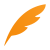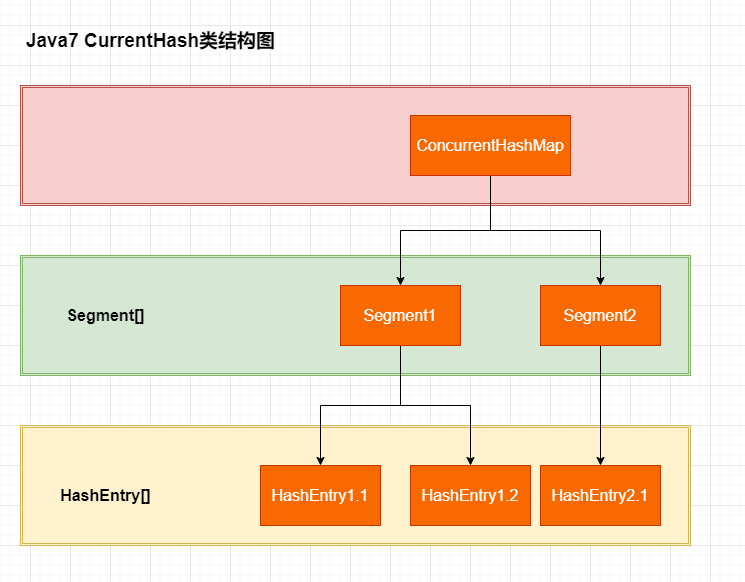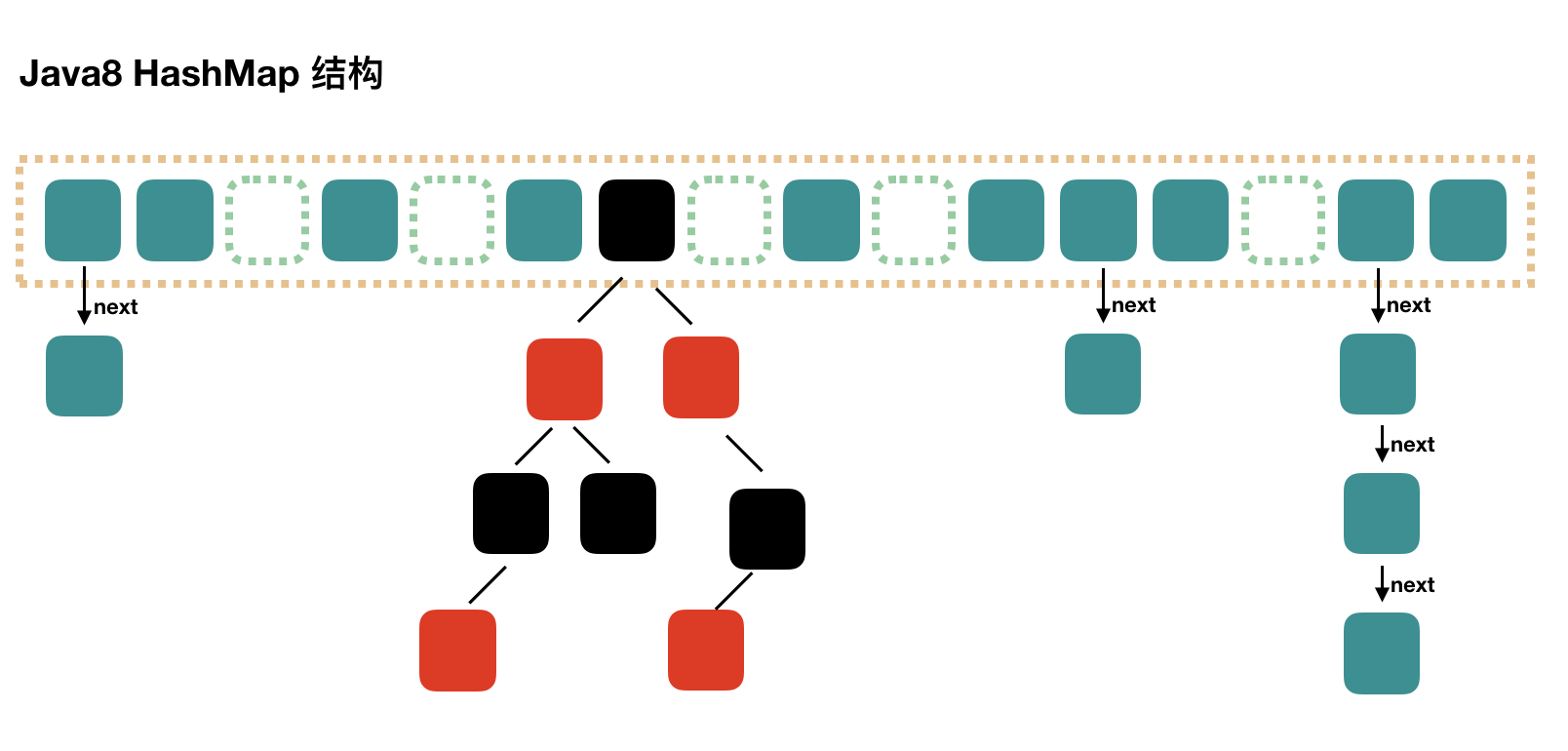# 死磕Java并发编程（8）：CurrentHashMap如何实现高效地线程安全？在Java8中有哪些设计实现的演进？2020 年 05 月 07 日 阅读数: 20## 为什么需要 ConcurrentHashMap？

static final HashMap<String, String> map = new HashMap<String, String>(2);Thread t = new Thread(new Runnable() {    @Override    public void run() {        for (int i = 0; i < 100000; i++) {            int finalI1 = i;            new Thread(new Runnable() {                @Override                public void run() {                    map.put(String.valueOf(finalI1), "");                }            }, "ftf" + i).start();        }    }}, "ftf");t.start();t.join();

## Java7中ConcurrentHashMap分析

• 分离锁，也就是将内部进行分段（Segment），里面则是 HashEntry 的数组，和 HashMap 类似，哈希相同的条目也是以链表形式存放。

• HashEntry 内部使用 volatile 的 value 字段来保证可见性，也利用了不可变对象的机制以改进利用 Unsafe 提供的底层能力，比如 volatile access，去直接完成部分操作，以最优化性能，毕竟 Unsafe 中的很多操作都是 JVM intrinsic 优化过的。### 初始化

ConcurrentHashMap 初始化方法是通过 initialCapacity 、loadFactor 和 concurrencyLevel 等几个参数来初始化segment数组、段偏移量 segmentShift 、段掩码 segmentMask 和每个 segment 里的 HashEntry 数组来实现的。

public ConcurrentHashMap(int initialCapacity,                         float loadFactor, int concurrencyLevel) {    if (!(loadFactor > 0) || initialCapacity < 0 || concurrencyLevel <= 0)        throw new IllegalArgumentException();    if (concurrencyLevel > MAX_SEGMENTS)        concurrencyLevel = MAX_SEGMENTS;    // Find power-of-two sizes best matching arguments    int sshift = 0;    int ssize = 1;    // 计算并行级别 ssize，因为要保持并行级别是 2 的 n 次方    while (ssize < concurrencyLevel) {        ++sshift;        ssize <<= 1;    }    // 我们这里先不要那么烧脑，用默认值，concurrencyLevel 为 16，sshift 为 4    // 那么计算出 segmentShift 为 28，segmentMask 为 15，后面会用到这两个值    this.segmentShift = 32 - sshift;    this.segmentMask = ssize - 1;    if (initialCapacity > MAXIMUM_CAPACITY)        initialCapacity = MAXIMUM_CAPACITY;    // initialCapacity 是设置整个 map 初始的大小，    // 这里根据 initialCapacity 计算 Segment 数组中每个位置可以分到的大小    // 如 initialCapacity 为 64，那么每个 Segment 可以分到 4 个    int c = initialCapacity / ssize;    if (c * ssize < initialCapacity)        ++c;    // 默认 MIN_SEGMENT_TABLE_CAPACITY 是 2，这个值也是有讲究的，因为这样的话，对于具体的槽上，插入一个元素不至于扩容，插入第二个的时候才会扩容    int cap = MIN_SEGMENT_TABLE_CAPACITY;     while (cap < c)        cap <<= 1;    // 创建 Segment 数组，    // 并创建数组的第一个元素 segment    Segment<K,V> s0 =        new Segment<K,V>(loadFactor, (int)(cap * loadFactor),                         (HashEntry<K,V>[])new HashEntry[cap]);    Segment<K,V>[] ss = (Segment<K,V>[])new Segment[ssize];    // 往数组写入 segment    UNSAFE.putOrderedObject(ss, SBASE, s0); // ordered write of segments    this.segments = ss;}

• Segment 数组长度为 16，不可以扩容

• Segment[i] 的默认大小为 2，负载因子是 0.75，得出初始阈值为 1.5，也就是以后插入第一个元素不会触发扩容，插入第二个会进行第一次扩容

• 这里初始化了 segment，其他位置还是 null，至于为什么要初始化 segment，后面的代码会介绍

• 当前段偏移量 segmentShift 的值为 32 - 4 = 28，段掩码 segmentMask 为 16 - 1 = 15，这两个值马上就会用到

### get 操作

get 操作需要保证的是可见性，所以并没有什么同步逻辑。

1. 计算 hash 值，找到 segment 数组中的具体位置

2. segment 中也是一个数组（HashEntry数组），根据 hash 找到数组中具体的位置

3. 到这里是链表了，HashEntry 是链表中的元素，顺着链表进行查找即可

public V get(Object key) {    Segment<K,V> s; // manually integrate access methods to reduce overhead    HashEntry<K,V>[] tab;    // 1. hash 值，32位    int h = hash(key);    // 利用位操作替换普通数学运算,将hash值无符号左移段偏移量位，即取高四位，在与上段掩码（15二进制位1111）    long u = (((h >>> segmentShift) & segmentMask) << SSHIFT) + SBASE;    // 2. 根据 hash 找到对应的 segment，利用Unsafe直接进行volatile access    if ((s = (Segment<K,V>)UNSAFE.getObjectVolatile(segments, u)) != null &&        (tab = s.table) != null) {        // 3. 找到segment 内部数组相应位置的链表，遍历        for (HashEntry<K,V> e = (HashEntry<K,V>) UNSAFE.getObjectVolatile                 (tab, ((long)(((tab.length - 1) & h)) << TSHIFT) + TBASE);             e != null; e = e.next) {            K k;            if ((k = e.key) == key || (e.hash == h && key.equals(k)))                return e.value;        }    }    return null;}

### put操作

public V put(K key, V value) {    Segment<K,V> s;    if (value == null)        throw new NullPointerException();    // 1. 二次哈希，以保证数据的分散性，避免哈希冲突 int hash = hash(key.hashCode());    // 2. 根据 hash 值找到 Segment 数组中的位置 j    //    hash 是 32 位，无符号右移 segmentShift(28) 位，剩下高 4 位，    //    然后和 segmentMask(15) 做一次与操作，也就是说 j 是 hash 值的高 4 位，也就是segment的数组下标    int j = (hash >>> segmentShift) & segmentMask;    // 刚刚说了，初始化的时候初始化了 segment，但是其他位置还是 null，    // ensureSegment(j) 对 segment[j] 进行初始化    if ((s = (Segment<K,V>)UNSAFE.getObject          // nonvolatile; recheck         (segments, (j << SSHIFT) + SBASE)) == null) //  in ensureSegment        s = ensureSegment(j);    // 3. 插入新值到 槽 s 中    return s.put(key, hash, value, false);}

final V put(K key, int hash, V value, boolean onlyIfAbsent) {    // 在往该 segment 写入前，需要先获取该 segment 的独占锁    //    先看主流程，后面还会具体介绍这部分内容    HashEntry<K,V> node = tryLock() ? null :        scanAndLockForPut(key, hash, value);    V oldValue;    try {        // 这个是 segment 内部的数组        HashEntry<K,V>[] tab = table;        // 再利用 hash 值，求应该放置的数组下标        int index = (tab.length - 1) & hash;        // first 是数组该位置处的链表的表头        HashEntry<K,V> first = entryAt(tab, index);        // 下面这串 for 循环虽然很长，不过也很好理解，想象当前位置链表不为空则先遍历找是否存在，如果存在则覆盖，否则放到合适的位置        for (HashEntry<K,V> e = first;;) {            if (e != null) {                K k;                if ((k = e.key) == key ||                    (e.hash == hash && key.equals(k))) {                    oldValue = e.value;                    if (!onlyIfAbsent) {                        // 覆盖旧值                        e.value = value;                        ++modCount;                    }                    break;                }                // 继续顺着链表走                e = e.next;            }            else {                // node 到底是不是 null，这个要看获取锁的过程，不过和这里都没有关系。                // 如果不为 null，那就直接将它设置为链表表头；如果是null，初始化并设置为链表表头。                if (node != null)                    node.setNext(first);                else                    node = new HashEntry<K,V>(hash, key, value, first);                int c = count + 1;                // 如果超过了该 segment 的阈值，这个 segment 需要扩容                if (c > threshold && tab.length < MAXIMUM_CAPACITY)                    rehash(node); // 扩容后面也会具体分析                else                    // 没有达到阈值，将 node 放到数组 tab 的 index 位置，                    // 其实就是将新的节点设置成原链表的表头                    setEntryAt(tab, index, node);                ++modCount;                count = c;                oldValue = null;                break;            }        }    } finally {        // 解锁        unlock();    }    return oldValue;}

### rehash：扩容操作

// 方法参数上的 node 是这次扩容后，需要添加到新的数组中的数据。private void rehash(HashEntry<K,V> node) {    HashEntry<K,V>[] oldTable = table;    int oldCapacity = oldTable.length;    // 2 倍    int newCapacity = oldCapacity << 1;    threshold = (int)(newCapacity * loadFactor);    // 创建新数组    HashEntry<K,V>[] newTable =        (HashEntry<K,V>[]) new HashEntry[newCapacity];    // 新的掩码，如从 16 扩容到 32，那么 sizeMask 为 31，对应二进制 ‘000...00011111’    int sizeMask = newCapacity - 1;    // 遍历原数组，老套路，将原数组位置 i 处的链表拆分到 新数组位置 i 和 i+oldCap 两个位置    for (int i = 0; i < oldCapacity ; i++) {        // e 是链表的第一个元素        HashEntry<K,V> e = oldTable[i];        if (e != null) {            HashEntry<K,V> next = e.next;            // 计算应该放置在新数组中的位置，            // 假设原数组长度为 16，e 在 oldTable 处，那么 idx 只可能是 3 或者是 3 + 16 = 19            int idx = e.hash & sizeMask;            // 该位置处只有一个元素，那比较好办,直接放到新数组中对应的位置            if (next == null)                   newTable[idx] = e;            else { // Reuse consecutive sequence at same slot                // e 是链表表头                HashEntry<K,V> lastRun = e;                // idx 是当前链表的头结点 e 的新位置                int lastIdx = idx;                // 下面这个 for 循环会找到一个 lastRun 节点，这个节点之后的所有元素是将要放到一起的                for (HashEntry<K,V> last = next;                     last != null;                     last = last.next) {                    int k = last.hash & sizeMask;                    if (k != lastIdx) {                        lastIdx = k;                        lastRun = last;                    }                }                // 将 lastRun 及其之后的所有节点组成的这个链表放到 lastIdx 这个位置                newTable[lastIdx] = lastRun;                // 下面的操作是处理 lastRun 之前的节点，                //    这些节点可能分配在另一个链表中，也可能分配到上面的那个链表中                for (HashEntry<K,V> p = e; p != lastRun; p = p.next) {                    V v = p.value;                    int h = p.hash;                    int k = h & sizeMask;                    HashEntry<K,V> n = newTable[k];                    newTable[k] = new HashEntry<K,V>(h, p.key, v, n);                }            }        }    }    // 将新来的 node 放到新数组中刚刚的 两个链表之一 的 头部    int nodeIndex = node.hash & sizeMask; // add the new node    node.setNext(newTable[nodeIndex]);    newTable[nodeIndex] = node;    table = newTable;}

Doug Lea 大神这块的想法一般人可能是想不到的，毕竟作为并发包中的基础类 都是为了将并发性能做到极致的。但是也有最差的情况，就是找到的 lastRun 是链表的最后一个元素，或者排在倒数，那么这次遍历就显得多余了，而且浪费了性能。不过 Doug Lea 也说了，根据统计，如果使用默认的阈值，大约只有 1/6 的节点需要克隆

## Java8中ConcurrentHashMap分析

• Java8 对 HashMap 进行了一些修改，最大的不同就是利用了红黑树，所以其由 数组+链表+红黑树 组成。

• 数据存储利用 volatile 来保证可见性。

• 使用 CAS 等操作，在特定场景进行无锁并发操作。Java7 中使用 HashEntry 来代表每个 HashMap 中的数据节点，Java8 中使用 Node，基本没有区别，都是 key，value，hash 和 next 这四个属性，不过，Node 只能用于链表的情况，红黑树的情况需要使用 TreeNode

static class Node<K,V> implements Map.Entry<K,V> { final int hash; final K key; volatile V val; volatile Node<K,V> next; // …}

### put操作

final V putVal(K key, V value, boolean onlyIfAbsent) {    if (key == null || value == null) throw new NullPointerException();    // 得到 hash 值    int hash = spread(key.hashCode());    // 用于记录相应链表的长度    int binCount = 0;    for (Node<K,V>[] tab = table;;) {        Node<K,V> f; int n, i, fh;        // 如果数组"空"，进行数组初始化        if (tab == null || (n = tab.length) == 0)            // 初始化数组            tab = initTable();        // 找该 hash 值对应的数组下标，得到第一个节点 f        else if ((f = tabAt(tab, i = (n - 1) & hash)) == null) {            // 如果数组该位置为空，            // 用一次 CAS 操作将这个新值放入其中即可，这个 put 操作差不多就结束了，可以拉到最后面            // 如果 CAS 失败，那就是有并发操作，进到下一个循环就好了            if (casTabAt(tab, i, null,                         new Node<K,V>(hash, key, value, null)))                break;                   // no lock when adding to empty bin        }        // hash 居然可以等于 MOVED，这个需要到后面才能看明白，不过从名字上也能猜到，肯定是因为在扩容        else if ((fh = f.hash) == MOVED)            // 帮助数据迁移，这个等到看完数据迁移部分的介绍后，再理解这个就很简单了            tab = helpTransfer(tab, f);        else { // 到这里就是说，f 是该位置的头结点，而且不为空            V oldVal = null;            // 获取数组该位置的头结点的监视器锁            synchronized (f) {                if (tabAt(tab, i) == f) {                    if (fh >= 0) { // 头结点的 hash 值大于 0，说明是链表                        // 用于累加，记录链表的长度                        binCount = 1;                        // 遍历链表                        for (Node<K,V> e = f;; ++binCount) {                            K ek;                            // 如果发现了"相等"的 key，判断是否要进行值覆盖，然后也就可以 break 了                            if (e.hash == hash &&                                ((ek = e.key) == key ||                                 (ek != null && key.equals(ek)))) {                                oldVal = e.val;                                if (!onlyIfAbsent)                                    e.val = value;                                break;                            }                            // 到了链表的最末端，将这个新值放到链表的最后面                            Node<K,V> pred = e;                            if ((e = e.next) == null) {                                pred.next = new Node<K,V>(hash, key,                                                          value, null);                                break;                            }                        }                    }                    else if (f instanceof TreeBin) { // 红黑树                        Node<K,V> p;                        binCount = 2;                        // 调用红黑树的插值方法插入新节点                        if ((p = ((TreeBin<K,V>)f).putTreeVal(hash, key,                                                       value)) != null) {                            oldVal = p.val;                            if (!onlyIfAbsent)                                p.val = value;                        }                    }                }            }            if (binCount != 0) {                // 判断是否要将链表转换为红黑树，临界值和 HashMap 一样，也是 8                if (binCount >= TREEIFY_THRESHOLD)                    // 这个方法和 HashMap 中稍微有一点点不同，那就是它不是一定会进行红黑树转换，                    // 如果当前数组的长度小于 64，那么会选择进行数组扩容，而不是转换为红黑树                    // 具体源码我们就不看了，扩容部分后面说                    treeifyBin(tab, i);                if (oldVal != null)                    return oldVal;                break;            }        }    }    //     addCount(1L, binCount);    return null;}

put 的主流程看完了，但是至少留下了几个问题，第一个是初始化，第二个是扩容，第三个是帮助数据迁移，这些我们都会在后面进行一一介绍。

### 初始化数组：initTable

private final Node<K,V>[] initTable() {    Node<K,V>[] tab; int sc;    while ((tab = table) == null || tab.length == 0) {        // 初始化的"功劳"被其他线程"抢去"了        if ((sc = sizeCtl) < 0)            Thread.yield(); // lost initialization race; just spin        // CAS 一下，将 sizeCtl 设置为 -1，代表抢到了锁        else if (U.compareAndSwapInt(this, SIZECTL, sc, -1)) {            try {                if ((tab = table) == null || tab.length == 0) {                    // DEFAULT_CAPACITY 默认初始容量是 16                    int n = (sc > 0) ? sc : DEFAULT_CAPACITY;                    @SuppressWarnings("unchecked")                    // 初始化数组，长度为 16 或初始化时提供的长度                    Node<K,V>[] nt = (Node<K,V>[])new Node<?,?>[n];                    // 将这个数组赋值给 table，table 是 volatile 的                    table = tab = nt;                    // 如果 n 为 16 的话，那么这里 sc = 12                    // 其实就是 0.75 * n                    sc = n - (n >>> 2);                }            } finally {                // 设置 sizeCtl 为 sc，我们就当是 12 吧                sizeCtl = sc;            }            break;        }    }    return tab;}

### 链表转为红黑树：treeifyBin

private final void treeifyBin(Node<K,V>[] tab, int index) {    Node<K,V> b; int n, sc;    if (tab != null) {        if ((n = tab.length) < MIN_TREEIFY_CAPACITY)            // 所以，如果数组长度小于 64 的时候，其实也就是 32 或者 16 或者更小的时候，会进行数组扩容            tryPresize(n << 1);        // b 是头结点        else if ((b = tabAt(tab, index)) != null && b.hash >= 0) {            // 加锁            synchronized (b) {                if (tabAt(tab, index) == b) {                    TreeNode<K,V> hd = null, tl = null;                    // 下面就是遍历链表，建立一颗红黑树                    for (Node<K,V> e = b; e != null; e = e.next) {                        TreeNode<K,V> p =                            new TreeNode<K,V>(e.hash, e.key, e.val,                                              null, null);                        if ((p.prev = tl) == null)                            hd = p;                        else                            tl.next = p;                        tl = p;                    }                    // 将红黑树设置到数组相应位置中                    setTabAt(tab, index, new TreeBin<K,V>(hd));                }            }        }    }}

### 扩容：tryPresize

// 首先要说明的是，方法参数 size 传进来的时候就已经翻了倍了private final void tryPresize(int size) {    // c：size 的 1.5 倍，再加 1，再往上取最近的 2 的 n 次方。    int c = (size >= (MAXIMUM_CAPACITY >>> 1)) ? MAXIMUM_CAPACITY :        tableSizeFor(size + (size >>> 1) + 1);    // 目前容器大小    int sc;    while ((sc = sizeCtl) >= 0) {        Node<K,V>[] tab = table; int n;        // 这个 if 分支和之前说的初始化数组的代码基本上是一样的，在这里，我们可以不用管这块代码        if (tab == null || (n = tab.length) == 0) {            n = (sc > c) ? sc : c;            if (U.compareAndSwapInt(this, SIZECTL, sc, -1)) {                try {                    if (table == tab) {                        @SuppressWarnings("unchecked")                        Node<K,V>[] nt = (Node<K,V>[])new Node<?,?>[n];                        table = nt;                        // 16-4=12                        sc = n - (n >>> 2);                    }                } finally {                    sizeCtl = sc;                }            }        }        // 小于目前大小或者达到最大值直接返回        else if (c <= sc || n >= MAXIMUM_CAPACITY)            break;        // 说明是tab过程中没有发生变化，类似于懒加载的双重检查        else if (tab == table) {            // value = 32795            int rs = resizeStamp(n);            if (sc < 0) {                Node<K,V>[] nt;                if ((sc >>> RESIZE_STAMP_SHIFT) != rs || sc == rs + 1 ||                    sc == rs + MAX_RESIZERS || (nt = nextTable) == null ||                    transferIndex <= 0)                    break;                // 2. 用 CAS 将 sizeCtl 加 1，然后执行 transfer 方法 此时 nextTab 不为 null                if (U.compareAndSwapInt(this, SIZECTL, sc, sc + 1))                    transfer(tab, nt);            }            // 1. 将 sizeCtl 设置为 (rs << RESIZE_STAMP_SHIFT) + 2)            // 我是没看懂这个值真正的意义是什么？不过可以计算出来的是，结果是一个比较大的负数            // 调用 transfer 方法，此时 nextTab 参数为 null            else if (U.compareAndSwapInt(this, SIZECTL, sc,                                         (rs << RESIZE_STAMP_SHIFT) + 2))                transfer(tab, null);        }    }}

### 数据迁移：transfer

private final void transfer(Node<K,V>[] tab, Node<K,V>[] nextTab) {    int n = tab.length, stride;    // stride 在单核下直接等于 n，多核模式下为 (n>>>3)/NCPU，最小值是 16    // stride 可以理解为”步长“，有 n 个位置是需要进行迁移的，    // 将这 n 个任务分为多个任务包，每个任务包有 stride 个任务    if ((stride = (NCPU > 1) ? (n >>> 3) / NCPU : n) < MIN_TRANSFER_STRIDE)        stride = MIN_TRANSFER_STRIDE; // subdivide range    // 如果 nextTab 为 null，先进行一次初始化    // 前面我们说了，外围会保证第一个发起迁移的线程调用此方法时，参数 nextTab 为 null    // 之后参与迁移的线程调用此方法时，nextTab 不会为 null    if (nextTab == null) {            // initiating        try {            @SuppressWarnings("unchecked")            // 容量翻倍            Node<K,V>[] nt = (Node<K,V>[])new Node<?,?>[n << 1];            nextTab = nt;        } catch (Throwable ex) {      // try to cope with OOME            sizeCtl = Integer.MAX_VALUE;            return;        }        // nextTable 是 ConcurrentHashMap 中的属性        nextTable = nextTab;        // transferIndex 也是 ConcurrentHashMap 的属性，用于控制迁移的位置        transferIndex = n;    }    int nextn = nextTab.length;    // ForwardingNode 翻译过来就是正在被迁移的 Node    // 这个构造方法会生成一个Node，key、value 和 next 都为 null，关键是 hash 为 MOVED    // 后面我们会看到，原数组中位置 i 处的节点完成迁移工作后，    // 就会将位置 i 处设置为这个 ForwardingNode，用来告诉其他线程该位置已经处理过了    // 所以它其实相当于是一个标志。    ForwardingNode<K,V> fwd = new ForwardingNode<K,V>(nextTab);    // advance 指的是做完了一个位置的迁移工作，可以准备做下一个位置的了    boolean advance = true;    boolean finishing = false; // to ensure sweep before committing nextTab    // 下面这个 for 循环，最难理解的在前面，而要看懂它们，应该先看懂后面的，然后再倒回来    // i 是位置索引，bound 是边界，注意是从后往前    for (int i = 0, bound = 0;;) {        Node<K,V> f; int fh;        // 下面这个 while 真的是不好理解        // advance 为 true 表示可以进行下一个位置的迁移了        // 简单理解为：i 指向了 transferIndex，bound 指向了 transferIndex-stride        while (advance) {            int nextIndex, nextBound;            if (--i >= bound || finishing)                advance = false;            // 将 transferIndex 值赋给 nextIndex            // 这里 transferIndex 一旦小于等于 0，说明原数组的所有位置都有相应的线程去处理了            else if ((nextIndex = transferIndex) <= 0) {                i = -1;                advance = false;            }            else if (U.compareAndSwapInt                     (this, TRANSFERINDEX, nextIndex,                      nextBound = (nextIndex > stride ?                                   nextIndex - stride : 0))) {                // 看括号中的代码，nextBound 是这次迁移任务的边界，注意，是从后往前                bound = nextBound;                i = nextIndex - 1;                advance = false;            }        }        if (i < 0 || i >= n || i + n >= nextn) {            int sc;            // 所有的迁移操作已经完成            if (finishing) {                nextTable = null;                // 将新的 nextTab 赋值给 table 属性，完成迁移                table = nextTab;                // 重新计算 sizeCtl：n 是原数组长度，所以 sizeCtl 得出的值将是新数组长度的 0.75 倍                sizeCtl = (n << 1) - (n >>> 1);                return;            }            // 之前我们说过，sizeCtl 在迁移前会设置为 (rs << RESIZE_STAMP_SHIFT) + 2            // 然后，每有一个线程参与迁移就会将 sizeCtl 加 1，            // 这里使用 CAS 操作对 sizeCtl 进行减 1，代表做完了属于自己的任务            if (U.compareAndSwapInt(this, SIZECTL, sc = sizeCtl, sc - 1)) {                // 任务结束，方法退出                if ((sc - 2) != resizeStamp(n) << RESIZE_STAMP_SHIFT)                    return;                // 到这里，说明 (sc - 2) == resizeStamp(n) << RESIZE_STAMP_SHIFT，                // 也就是说，所有的迁移任务都做完了，也就会进入到上面的 if(finishing){} 分支了                finishing = advance = true;                i = n; // recheck before commit            }        }        // 如果位置 i 处是空的，没有任何节点，那么放入刚刚初始化的 ForwardingNode ”空节点“        else if ((f = tabAt(tab, i)) == null)            advance = casTabAt(tab, i, null, fwd);        // 该位置处是一个 ForwardingNode，代表该位置已经迁移过了        else if ((fh = f.hash) == MOVED)            advance = true; // already processed        else {            // 对数组该位置处的结点加锁，开始处理数组该位置处的迁移工作            synchronized (f) {                if (tabAt(tab, i) == f) {                    Node<K,V> ln, hn;                    // 头结点的 hash 大于 0，说明是链表的 Node 节点                    if (fh >= 0) {                        // 下面这一块和 Java7 中的 ConcurrentHashMap 迁移是差不多的，                        // 需要将链表一分为二，                        // 找到原链表中的 lastRun，然后 lastRun 及其之后的节点是一起进行迁移的                        // lastRun 之前的节点需要进行克隆，然后分到两个链表中                        int runBit = fh & n;                        Node<K,V> lastRun = f;                        for (Node<K,V> p = f.next; p != null; p = p.next) {                            int b = p.hash & n;                            if (b != runBit) {                                runBit = b;                                lastRun = p;                            }                        }                        if (runBit == 0) {                            ln = lastRun;                            hn = null;                        }                        else {                            hn = lastRun;                            ln = null;                        }                        for (Node<K,V> p = f; p != lastRun; p = p.next) {                            int ph = p.hash; K pk = p.key; V pv = p.val;                            if ((ph & n) == 0)                                ln = new Node<K,V>(ph, pk, pv, ln);                            else                                hn = new Node<K,V>(ph, pk, pv, hn);                        }                        // 其中的一个链表放在新数组的位置 i                        setTabAt(nextTab, i, ln);                        // 另一个链表放在新数组的位置 i+n                        setTabAt(nextTab, i + n, hn);                        // 将原数组该位置处设置为 fwd，代表该位置已经处理完毕，                        // 其他线程一旦看到该位置的 hash 值为 MOVED，就不会进行迁移了                        setTabAt(tab, i, fwd);                        // advance 设置为 true，代表该位置已经迁移完毕                        advance = true;                    }                    else if (f instanceof TreeBin) {                        // 红黑树的迁移                        TreeBin<K,V> t = (TreeBin<K,V>)f;                        TreeNode<K,V> lo = null, loTail = null;                        TreeNode<K,V> hi = null, hiTail = null;                        int lc = 0, hc = 0;                        for (Node<K,V> e = t.first; e != null; e = e.next) {                            int h = e.hash;                            TreeNode<K,V> p = new TreeNode<K,V>                                (h, e.key, e.val, null, null);                            if ((h & n) == 0) {                                if ((p.prev = loTail) == null)                                    lo = p;                                else                                    loTail.next = p;                                loTail = p;                                ++lc;                            }                            else {                                if ((p.prev = hiTail) == null)                                    hi = p;                                else                                    hiTail.next = p;                                hiTail = p;                                ++hc;                            }                        }                        // 如果一分为二后，节点数少于 8，那么将红黑树转换回链表                        ln = (lc <= UNTREEIFY_THRESHOLD) ? untreeify(lo) :                            (hc != 0) ? new TreeBin<K,V>(lo) : t;                        hn = (hc <= UNTREEIFY_THRESHOLD) ? untreeify(hi) :                            (lc != 0) ? new TreeBin<K,V>(hi) : t;                        // 将 ln 放置在新数组的位置 i                        setTabAt(nextTab, i, ln);                        // 将 hn 放置在新数组的位置 i+n                        setTabAt(nextTab, i + n, hn);                        // 将原数组该位置处设置为 fwd，代表该位置已经处理完毕，                        // 其他线程一旦看到该位置的 hash 值为 MOVED，就不会进行迁移了                        setTabAt(tab, i, fwd);                        // advance 设置为 true，代表该位置已经迁移完毕                        advance = true;                    }                }            }        }    }}

## 总结

（全文完）fighting！

1. 周志明：《深入理解 Java 虚拟机》

2. 方腾飞：《Java 并发编程的艺术》